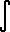Dave's Math Tables: Integral tanh(x)(Math | Calculus | Integrals | Table Of | tanh x)

 Discussion oftanh x dx = ln (cosh x) + C.

## 1. Proof

Strategy: Use definition of tanh; Use Substitution.  tanh x = sinh x  cosh x = (ex - e-x) / 2  (ex + e-x) / 2tanh x dx =ex - e-x  ex + e-x dx

set
u = ex + e-x
then we find
du = (ex - e-x) dx

substitute du= (ex - e-x) dx, u = ex + e-x
 =du  u

solve

= ln |u| + C

substitute back u = ex + e-x

= ln |ex + e-x| + C

since ex and e-x are always positive

= ln (ex + e-x) + C

since (ex + e-x)/2 = cosh(x)

= ln (2 cosh x) + C
= ln 2 + ln (cosh x) + C

ln 2 is merely a constant that can be combined with C

= ln (cosh x) + C
Q.E.D.